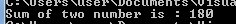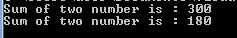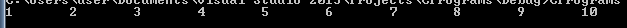# Scope Rules in C Programming

Scope of a variable is the visibility of that variable within the program or within function or block. C allows us to declare variables anywhere in the program. Unlike other programming language we need not declare them at the beginning of the program. Because of this feature, developer need not know all the variables that are required for the program. He can declare them whenever necessary. But the problem with this type of declare is that their life span. Developer should be aware of their life period within program or where he declares it. This is called scope of the variable.

Consider a program to find the sum of two numbers. We can write this program in different ways: using single main program, by declaring the variables at the point of access, by using function within the program etc.

```//Method 1
#include
void main(){
int intNum1, intNum2;
int intResult;

intNum1 = 50;
intNum2 = 130;

intResult = intNum1 + intNum2;

printf("Sum of two number is : %d", intResult);
}```In this method, all the variables that are accessed in the program are declared at the beginning of the main function. This is the traditional way of declaring the variables. These variables are available to access to any expression or statements throughout the main function. These variables cannot be accessed by any other function or block in the program or other program. The variables declared within the function or any block is called local variable to that function or block. That means scope of the local variables are limited to the function or block in which it is being declared and exists till the end of the function or block where it is declared. Whenever a local variable is declared, it is not automatically initialized. We need to explicitly assign value to it.

```//Method 2
#include
void main(){
int intNum1, intNum2;

intNum1 = 50;
intNum2 = 130;

int intResult = intNum1 + intNum2;

printf("Sum of two number is : %d", intResult);
}```In this method, result variable is declared when sum is calculated in the main program. Hence intResult comes into existence after it is being declared. If we try to access intResult before it is being declared, then the program will throw an error saying that intResult is not declared. Once it is declared, it can be accessed till the end of the main function. That means scope of the variable exists till the end of the block it is being declared. At the same time, intNum1 and intNum2 are declared at the beginning of the main function and can be accessed throughout the program.

```//Method 3
#include

int intResult; // Global variable

void main(){
int intNum1, intNum2;

intNum1 = 50;
intNum2 = 130;

intResult = intNum1 + intNum2;

printf("Sum of two number is : %d", intResult);
}```Here the variable intResult is declared outside the main function. It is not present in any other function or block. Hence it is called as global variable. Since this variable is not inside any block or function, it can be accessed by any function, block or expression. Hence the scope of global variable is not limited to any function or block; but it can be accessed by any function or block within the program. Global variables are initialized to the initial value defined for its datatype.

Below is another method of adding two numbers. Here a separate function is written to add and display the result. Two local variables of main function – intNum1 and intNum2 are passed to the function. Since it is local variable, it cannot be accessed by any other functions in the program. Since it passed to the function, these variables can be accessed by the function AddNum (). But here we need to note the difference between the variables in the main function and AddNum function. The variables in the main function are local to it and can be accessed by it alone. These local parameters are passed to AddNum function, and are local to this function now. These parameters of function are called as formal parameter of a function. It can have same name as variables passed from the calling function or different. Suppose we have same name for local variable of main function and formal parameter. In this case, compiler considers both of them as different variables even though they have same name and value.  Here memory address of variables at main function and AddNum function are different. This type of passing variable is called pass by value.

Below we have given different names in both the functions. Even though they are having same value and same variable is passed to the function, both of them cannot be accessed in other functions. For example, if we try to access intNum1 and intNum2 in AddNum function, it will throw an error. Similarly, intResult is a local variable of AddNum and can be accesses only within it

```//Method 4
#include

void main(){
int intNum1, intNum2;

intNum1 = 50;
intNum2 = 130;

//	printf("Sum of two number is : %d", intResult);
}

int intResult;

intResult = a + b;

printf("Sum of two number is : %d", intResult);
}```Suppose we declare intResult as global variable. Then we can access it in either main function or in AddNum function. It will contain the value based on the last expression that is being evaluated. Consider the below program where intResult is declared as global variable. It can now be accessed from any function. It contains the value depending on the expression being evaluated in that function.

```//Method 5
#include

int intResult;

void main(){
int intNum1=100, intNum2 =200;

intNum1 = 50;
intNum2 = 130;

intResult = intNum1 + intNum2;
printf("Sum of two number is : %d\n", intResult);
}

intResult = a + b;

printf("Sum of two number is : %d\n", intResult);
}```From all these examples, we understood that local variables are accessed only within the function or block it is being declared, whereas global variables are accessed throughout the program.

Consider a block of code below:

```//Method 6
#include

void main(){
int intArr = { 1, 2, 3, 4, 5, 6, 7, 8, 9, 10 };
for (int index =0, index<10, index++)
printf("%d\t", intArr[index]);

//printf("\nIndex = %d\n", index); // this row will throw an error
}```Here index variable is declared inside the ‘for’ loop. Even though index is inside the main function, it is declared inside a block in the main function. That means even though index is a local variable, for loop is considered as block and variables declared within it is local to it. Hence we can access index inside the for loop (need not be single line – it can have more than one line), but it cannot be accessed outside the loop – outside the block. The scope or life span of the variable index expires as soon as for loop ends – end of block.

Translate »